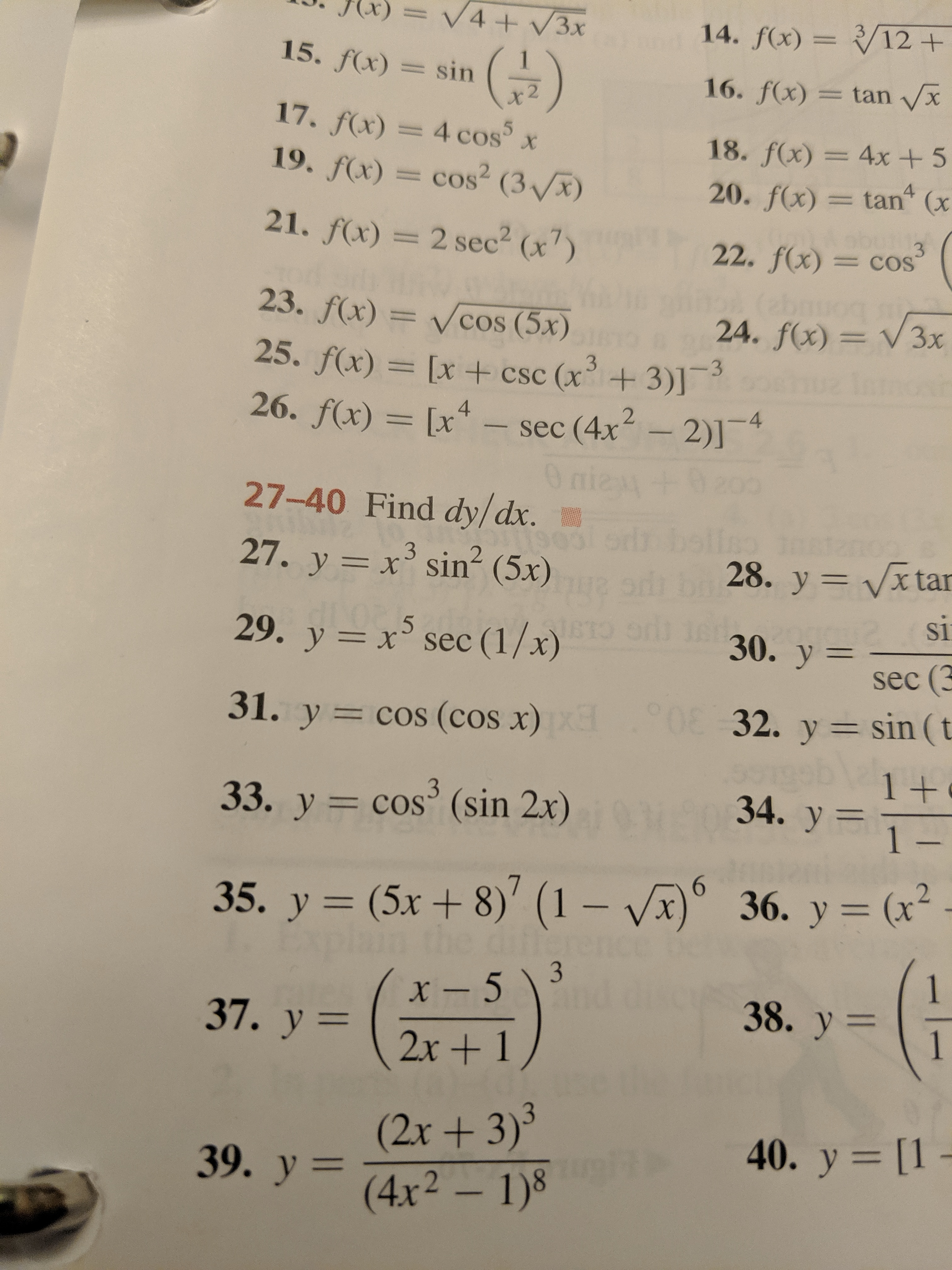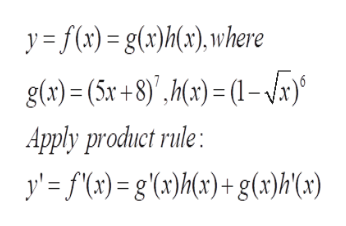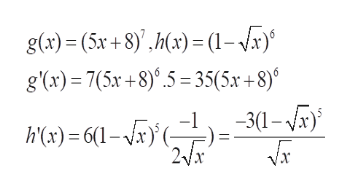V4+/3x14. f(x)=/12 +15. f(x)sin16. f(x)= tan xx 2fx) = 4 cos5x.f(x) = cos2 ( 318. f(x)= 4x + 5tan (x420. f(x)01 121. f(x)= 2 sec2 (x)322. f(x)=COSabr24. f(x) V3x23. f(x)=Vcos (5x)25. f(x) = [x +csc (x+3)]26. f(x) [xsec (4x 2)]4O nie +227-40 Find dy/dx.27. y x sin2 (5x)28. y Vx tarXsi30. y=.529. y x sec (1/x)sec (3032. y sin (t31. y cos (cos x)1 +34. y 1-333. y = cos (sin 2x)636. у 3 (х*36. y= (x(5x + 8)' (1 - Vx35. y1x-51338. y37. у —2x+1(2x+ 3)339. y4x2 1)40. y [1 -

Question

#35help_outlineImage TranscriptioncloseV4+/3x 14. f(x)=/12 + 15. f(x) sin 16. f(x)= tan x x 2 fx) = 4 cos5x .f(x) = cos2 ( 3 18. f(x)= 4x + 5 tan (x 4 20. f(x) 01 1 21. f(x) = 2 sec2 (x) 3 22. f(x) =COS abr 24. f(x) V3x 23. f(x)= Vcos (5x) 25. f(x) = [x +csc (x+3)] 26. f(x) [x sec (4x 2)]4 O nie + 2 27-40 Find dy/dx. 27. y x sin2 (5x) 28. y Vx tar X si 30. y= .5 29. y x sec (1/x) sec (3 032. y sin (t 31. y cos (cos x) 1 + 34. y 1- 3 33. y = cos (sin 2x) 6 36. у 3 (х* 36. y= (x (5x + 8)' (1 - Vx 35. y 1 x-513 38. y 37. у — 2x+1 (2x+ 3)3 39. y4x2 1) 40. y [1 - fullscreen
Step 1

35) to differentiate the given function

Step 2help_outlineImage Transcriptionclosey=f(x)=g(x)h(), where g(a)= (5x+8)',h(x)= (1-x) Apply product rule: y'f(x)= g'(ax)M{t)+g(»)h(cx) fullscreen
Step 3

Apply chain rule to differe...help_outlineImage Transcriptioncloseg(x)= (5x + 8),h) (1-x) g(x) 7(5r+8).5 35(5x +8) hc) = 6(-r}(-}}=30-a 2Vx Vx fullscreen

Want to see the full answer?

See Solution

Want to see this answer and more?

Our solutions are written by experts, many with advanced degrees, and available 24/7

See Solution
Tagged in

Derivative